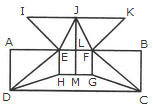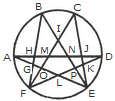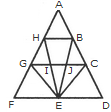# Logical Reasoning and Analytical Ability - Online Test

Q1. Find the number of triangles in the given figure.Explaination / Solution:

The figure may be labelled as shown.The simplest triangles are AHG, AIG, AIB, JFE, CJE and CED i.e. 6 in number.

The triangles composed of two components each are ABG, CFE, ACJ and EGI i.e. 4 in number.

The triangles composed of three components each are ACE, AGE and CFD i.e. 3 in number.

There is only one triangle i.e. AHE composed of four components.

Therefore, There are 6 + 4 + 3 + 1 = 14 triangles in the given figure.

Q2.
Directions: Below is given a passage followed by several possible inferences which can be drawn from the facts stated in the passage. You have to examine each inference separately in the context of the passage and decide upon its degree of truth or falsity.
Note: Each of the five questions has only one distinct answer, ie, no two questions can have the same answer. If
you get the same answer for more than one question, consider both again and decide which one of the two would more definitely be that answer and in the same way review the others also.
Cardiovascular disease is so prevalent that virtually all businesses are likely to have employees who suffer from,
or may develop, this condition. Research shows that between 50-80% of all people who suffer a heart attack are able to return to work. However, this may not be possible if they have previously been involved in heavy physical work. In such cases, it may be possible to move the employee to lighter duties, with appropriate retraining where necessary. Similarly Difficult pressure, stressful work, even where it does not involve physical activity, should also be avoided. Human resource managers should be aware of the implications of job roles for employees with a cardiac condition.
Employees who suffer from cardiovascular diseases are mostly
Explaination / Solution:

Research- to work, Line shows that given statement is definitely false.

Q3. Find the minimum number of straight lines required to make the given figure.Explaination / Solution:

The figure may be labelled as shown.The horizontal lines are IK, AB, HG and DC i.e. 4 in number.

The vertical lines are AD, EH, JM, FG and BC i.e. 5 in number.

The slanting lines are IE, JE, JF, KF, DE, DH, FC and GC i.e. 8 is number.

Thus, there are 4 + 5 + 8 = 17 straight lines in the figure.

Q4. Employees suffering from cardiovascular diseases are unable to handle stressful situations.
Explaination / Solution:

Similarly- be avoided. Shows that given statement is probably true.

Q5. Find the number of triangles in the given figure.Explaination / Solution:

The figure may be labelled as shown.The simplest triangles are AGH, GFO, LFO, DJK, EKP, PEL and IMN i.e. 7 in number.

The triangles having two components each are GFL, KEL, AMO, NDP, BHN, CMJ, NEJ and HFM i.e. 8 in number.

The triangles having three components each are IOE, IFP, BIF and CEI i.e. 4 in number.

The triangles having four components each are ANE and DMF i.e. 2 in number.

The triangles having five components each are FCK, BGE and ADL i.e. 3 in number.

The triangles having six components each are BPF, COE, DHF and AJE i.e. 4 in number.

Total number of triangles in the figure = 7 + 8 + 4 + 2 + 3 + 4 = 28.

Q6. Heart disease can affect employee in any type of business.
Explaination / Solution:

Cardiovascular condition line shows that given statement is definitely true.

Q7. Find the number of triangles in the given figure.Explaination / Solution:

The figure may be labelled as shown.The simplest triangles are AHB, GHI, BJC, GFE, GIE, IJE, CEJ and CDE i.e. 8 in number.

The triangles composed of two components each are HEG, BEC, HBE, JGE and ICE i.e. 5 in number.

The triangles composed of three components each are FHE, GCE and BED i.e. 3 in number.

There is only one triangle i.e. AGC composed of four components.

There is only one triangle i.e. AFD composed of nine components.

Thus, there are 8 + 5 + 3 + 1 + 1 = 18 triangles in the given figure.

Q8. Direction: Below is given a passage followed by several possible inferences which can be drawn from the facts stated in the passage. You have to examine each inference separately in the context of the passage and decide upon its degree of truth or falsity.
In its most ambitious bid ever to house 6-crore slum dwellers and realize the vision of a slum free India, the government is rolling out a massive plan to build 50 lakh dwelling units in five years across 400 towns and cities. The program could free up thousands of acres of valuable government land across the country and generate crores worth of business for real estate developers. Proliferation of slums has had an adverse impact on the GOP growth for years. Slum dwellers are characterized by low productivity and susceptible to poor health conditions. The government believes that better housing low productivity and susceptible to poor health conditions. The government believes that better housing facilities will address social issues and also have a multiplier effect and serve as an economic stimulus.

Inference: Health and sanitary conditions in slums are far below the acceptable norm of human habitat in Indian cities and towns.
Explaination / Solution:

Consider the following line of the passage: “Slum dwellers are characterized by low productivity and susceptible to poor health conditions.” It is definitely true,

Q9. Find the number of triangles in the given figure.Explaination / Solution:

The figure may be labelled as shown.The simplest triangles are IJO, BCJ, CDK, KQL, MLQ, GFM, GHN and NIO i.e. 8 in number.

The triangles composed of two components each are ABO, AHO, NIJ, IGP, ICP, DEQ, FEQ, KLM, LCP and LGP i.e.10 in number.

The triangles composed of four components each are HAB, DEF, LGI, GIC, ICL and GLC i.e. 6 in number.

Total number of triangles in the figure = 8 + 10 + 6 = 24.

Q10. Direction: Below is given a passage followed by several possible inferences which can be drawn from the facts stated in the passage. You have to examine each inference separately in the context of the passage and decide upon its degree of truth or falsity.
Cities and towns of developed countries are free from slums.
Explaination / Solution:

There is no information about the developed countries. From the facts given in passage cannot say whether the inference is likely to be true or false. Hence, Option C is correct.